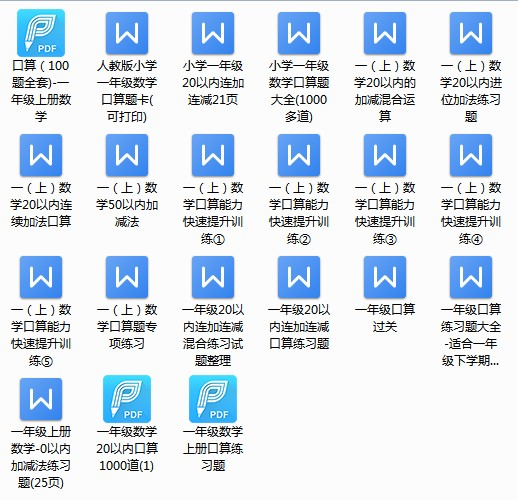8+0=    0+8=    0+3=   5+3=    2+7=    4+3=    2+7=    4+4=    6+1=   2+6=     3+4=    5+5=    4+4=    0+6=    6+4=     2+1=    3+2=    0+9=   4+4=    2+3=   3+4=    4+1=   1+5=    6+4=    0+3=     3+7=    9+1=    0+0=    0+3=    5+4=    8+1=    2+8=    8+2=    7+2=    3+7=    4+3=    4+4=    5+3=    1+2=    2+4=    5+5=    4+2=    8+1=    8+2=    2+7=    3+6=    6+1=    0+6=    0+5=    4+4=    2+8=    6+4=    4+4=    7+2=    9+1=    0+4=    3+4=    3+1=    6+3=    3+4=    7+0=    8+2=• 终生VIP优惠券
50

满100元可用

仅限购买永久VIP使用

有效期至2023-10-15 10:37

• 20元通用优惠券
20

满50元可用

仅限购买VIP使用

有效期至2023-10-06 11:01